Next: The Kalman-filter model Up: Model-based Approaches Previous: Model-based Approaches

## Snakes: an active model

Kass developed a novel technique for image segmentation, which was able to solve a large class of segmentation problems that had eluded more conventional techniques. He was interested in developing a model-based technique that could recognize familiar objects in the presence of noise and other ambiguities. Kass  proposed the concept of a snake, which was an active contour model using ``an energy minimizing spline guided by external constraint forces and influenced by image forces that pull it toward features such as lines and edges.'' Snakes have been successful in performing tasks such as edge detection (both actual as well as subjective), corner detection, motion tracking, and stereo matching.

A spline is nothing more than a polynomial or set of polynomials used to describe or approximate curves and surfaces. Although the polynomials that make up the spline can be of arbitrary degree, the most commonly used are cubic polynomials. For example, a simple two-dimensional curve can be approximated by the following pair of cubic equations: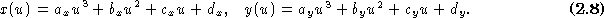Higher-order polynomials can have undesirable non-local properties. Complex shapes can be decomposed into smaller regions having fewer inflection points by the introduction of appropriately placed knots (i.e., control points). In equation, the variable u is called the spline parameter and typically varies over the interval [0,1]. Because cubic polynomials can have a maximum of two inflection points, complex shapes need to be decomposed into simpler segments or patches having at most two inflections. Although the coefficients a, b, c, d uniquely determine the shape of the spline, they are not usually specified directly. Instead, they are computed from other constraints such as continuity of zeroth, first- and second-order derivatives at boundary points between neighboring polynomial segments.

Snakes fall into the category of active contour models because they dynamically alter their shape and position while trying to seek a minimal energy state. A two-dimensional dynamic contour called v can be defined in terms of its x and y coordinates, which in turn are parameterized by s , the linear parameter, and t , the time parameter,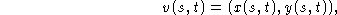where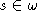, usually defined as the closed interval [0,1], and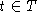, usually defined as the half-open interval [0,). The coefficients that minimize the energy of the spline can be found using either optimization techniques or differential calculus.

In Kass's original model, the total energy of a snake is made up of three subterms: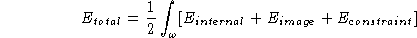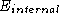is the internal energy of the spline and depends solely on the shape of the spline.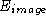is the image energy and depends solely on the image intensity values along the path of the spline.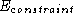is the constraint energy and is created by artificial energy fields imposed by the user or the high-level control agent. Other energy terms can be defined, but for the purpose of this review section, I will limit the discussion to these three terms.

The internal energy is defined as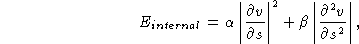wherecontrols the amount of stretching the snake is willing to undergo and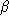controls the amount of flexing it will allow. Large values ofwill increase the internal energy of the snake as it stretches more and more, whereas small values ofwill make the energy function insensitive to the amount of stretch. Similarly, large values ofwill increase the internal energy of the snake as it develops more curves, whereas small values ofwill make the energy function insensitive to curves in the snake. Smaller values of bothandwill place fewer constraints on the size and shape of the snake.

The image energy of the snake is defined as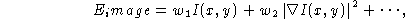where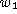is called the line coefficient and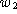is called the edge coefficient. Large positive values oftend to make the snake align itself with dark regions in the image, I(x,y), whereas large negative values oftend to make the snake align itself with bright regions in the image. Small absolute values ofmake the snake more indifferent to intensity variations in the image. Similarly, large positive values oftend to make the snake align itself with sharp edges in the image whereas large negative values ofmake the snake avoid these edges. Small absolute values ofmake the snake indifferent to edges in the image.

The external constraint energy is defined as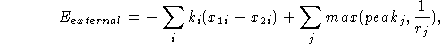where the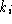terms are external spring factors and the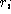terms are called volcano factors. A large value ofmakes the snake behave as if there was a powerful spring connected between a point on the image at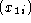and a point on the snake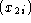. The larger the value of, the more powerful the force of the spring. Theterms are called volcanoes because the plot of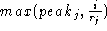resembles the profile of a symmetric volcano. Computationally, these volcano terms act as a repulsion force between a point on the image at a distance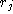from a point on the snake; the larger the value of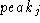, the stronger the force of repulsion. As a result, springs and volcanoes are a way of capturing high-level knowledge about images and the features contained within these images.

Using the internal and image energy forces, a snake will find desired image features in an autonomous fashion. The spring forces can be set up interactively by the user to confine the region in which a snake will operate. The volcano forces can be set up by the user to define regions that the snake should avoid.

Snakes have several advantages over classical feature extraction techniques:

• They can be controlled interactively by using appropriately placed springs and volcanoes.
• They are easy to manipulate because the external image forces behave in an intuitive manner.
• They are autonomous and self-adapting in their search for a minimal energy state.
• They can be made sensitive to image scale by incorporating Gaussian smoothing in the image energy function.
• They are relatively insensitive to noise and other ambiguities in the images because the integral operator is an inherent noise filter.
• They can be used to track dynamic objects in temporal as well as the spatial dimensions.

Snakes are not without their drawbacks, chief among which are the following:

• They can often get stuck in local minima states; this may be overcome by using simulated annealing techniques at the expense of longer computation times.
• They often overlook minute features in the process of minimizing the energy over the entire path of their contours.
• Their accuracy is governed by the convergence criteria used in the energy minimization technique; higher accuracies require tighter convergence criteria and hence, longer computation times.

In summary, snakes are a model-driven approach for solving many image understanding problems that are difficult, if not impossible, to tackle using classical approaches. Just like human vision, snakes start with an a priori model of what an object should look like. By using the smoothness constraints of the splines, they are able to fill in for missing and noisy boundary information. As a result, they are more robust than non-model based methods, which make little use of image structure. Much of the current research in active contour models deals with generalizing the form of the contours and overcoming the convergence and stability problems encountered during the energy minimization process     .Next: The Kalman-filter model Up: Model-based Approaches Previous: Model-based Approaches

Ramani Pichumani
Mon Jul 7 10:34:23 PDT 1997